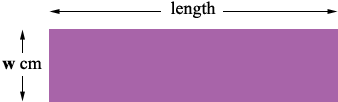SEARCH HOMEMath Central Quandaries & QueriesQuestion from shericka, a parent: a rectangle has a perimeter of 60cm. its length is 5 times greater than its width. what is its length?Hi,

I think the problem should say "a rectangle has a perimeter of 60cm. its length is 5 times its width. what is its length?"

Draw a diagram. (My diagram is not to scale.)Suppose the width is w cm. The length is 5 times the width so what is the length? The perimeter is the distance all the way around the rectangle. What is that? This distance is 60 cm. Solve for w.

PennyMath Central is supported by the University of Regina and The Pacific Institute for the Mathematical Sciences.